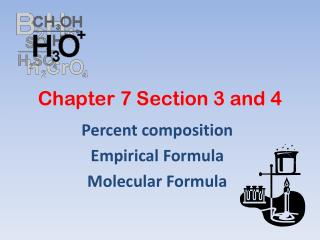Download PresentationChapter 7 Section 3 and 4

# Chapter 7 Section 3 and 4 - PowerPoint PPT PresentationDownload Presentation## Chapter 7 Section 3 and 4

- - - - - - - - - - - - - - - - - - - - - - - - - - - E N D - - - - - - - - - - - - - - - - - - - - - - - - - - -
##### Presentation Transcript

1. Chapter 7 Section 3 and 4 Percent composition Empirical Formula Molecular Formula

2. Calculating Formula Mass Calculate the formula mass of magnesium carbonate, MgCO3. 24.31 g + 12.01 g + 3(16.00 g) = 84.32 g

3. Calculating Percentage Composition Calculate the percentage composition of magnesium carbonate, MgCO3. From previous slide: 24.31 g + 12.01 g + 3(16.00 g) = 84.32 g 100.00

4. Formulas • Empirical formula: the smallest whole number ratio of atoms in a compound. • molecular formula = (empirical formula)nn = whole # • empirical formula = CH • molecular formula = = (CH)6 C6H6 • Molecular formula: the true number of atoms of each element in the formula of a compound.

5. Formulas(continued) Formulas for ionic compounds are ALWAYS empirical (lowest whole number ratio). Examples: NaCl MgCl2 Al2(SO4)3 K2CO3

6. Formulas(continued) Formulas for molecular compoundsMIGHT be empirical (lowest whole number ratio). Molecular: C6H12O6 H2O C12H22O11 Empirical: H2O CH2O C12H22O11

7. Empirical Formula Determination • Use the % composition of each element out of 100 grams or given mass of each element in grams. • Convert the mass of each element to moles using molar mass. • Divide each value of moles by the smallest of the values to give the smallest whole # ratio of moles. • This should give the subscripts for the formula in the smallest whole numbers. If after dividing, the answer is not a whole number , multiply all by 2 or 3 to get a whole numbers.

8. An unknown gas is found to be 24.0% carbon and 76.0% fluorine by mass. Determine the empirical formula of this gas. • 24.0g C x 1 mol = 2.00 mol 12.01 g C • 76.0 g F x 1 mol = 4.00 mol 19.00 g F 2.00/2.00 = 1 4.00/2.00 = 2 Multiply symbols by small whole # = C1F2or CF2 Work #2 on handout!

9. Empirical Formula Determination A certain acid contains 49.32% C, 43.84% O, and 6.85% H by mass. What is the empirical formula of the acid?

10. Empirical Formula Determination(part 2) Divide each value of moles by the smallest of the values. Carbon: Hydrogen: Oxygen:

11. Empirical Formula Determination(part 3) Multiply each number by an integer to obtain all whole numbers. Carbon: 1.50 Hydrogen: 2.50 Oxygen: 1.00 x 2 x 2 x 2 3 5 2 C3H5O2 Empirical formula: Work remaining problems on handout!

12. Summary Steps: • Changes masses to moles • Divided each by the smallest mole # • Establish a whole number ratio

13. Finding the Molecular FormulaDefinition – the true formula of a molecular compound. Sometimes called the “TRUE” formula! • Steps: • 1. Find the empirical formula. • 2. Divide molecular formula mass by empirical formula mass……MF/EF. • 3. Write molecular formula. The empirical formula for adipic acid is C3H5O2. The molecular mass of adipic acid is 146 g/mol. What is the molecular formula of adipic acid? 1. Find the formula mass of C3H5O2 3(12.01 g) + 5(1.01) + 2(16.00) = 73.08 g

14. Finding the Molecular Formula The empirical formula for adipic acid is C3H5O2. The molecular mass of adipic acid is 146 g/mol. What is the molecular formula of adipic acid? 2. Divide the molecular mass by the mass given by the emipirical formula. 3(12.01 g) + 5(1.01) + 2(16.00) = 73.08 g

15. Finding the Molecular Formula The empirical formula for adipic acid is C3H5O2. The molecular mass of adipic acid is 146 g/mol. What is the molecular formula of adipic acid? 3. Multiply the empirical formula by this number to get the molecular formula. 3(12.01 g) + 5(1.01) + 2(16.00) = 73.08 g (C3H5O2) x 2 = C6H10O4

16. Summary Steps for finding Molecular Formula: • Find the EF • Divide the given mass by the EF mass to create a ratio • Multiple the formula by the ratio # Work remaining problems on handout!

17. Hydrates • Hydrates: ionic compounds with water incorporated into their structure • Ex. CuSO4•5H2O cupric sulfate pentahydrate C. Solve like an EF problem